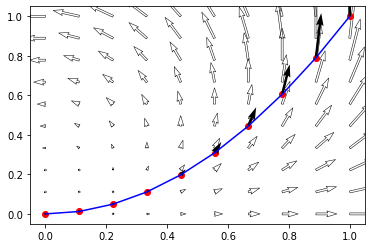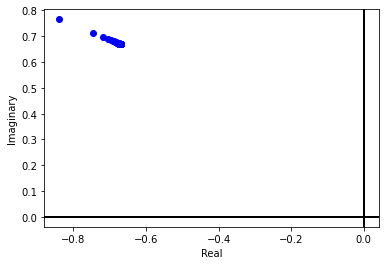# Contour Integration

A contour is a finite collection of paths joined end to end. A path can be represented as a continuous function form an closed iterval to $$\mathbb{C}$$ as follows

$\gamma: [a~ b]\subset \mathbb{R} \to \mathbb{C}; \quad \gamma = \gamma(t)$

Then the integration of any complex function along this path is is given by

$\int_\gamma f(z)dz = \int_a^b f(t)~\gamma'(t)~dt = \sum_{i=1}^n f(t_i) \left[\gamma(t_i)-\gamma(t_{i-1})\right]$

Question: Find the integration of $$f(z)=z^2$$ along the parabolic path from $$0$$ to $$1$$.

Answer: In order to find the integration we have to find to parametrize the curve as $$\gamma(t)=t+t^2i$$, $$t\in [0,1]$$.## Numerical Computation¶

We can subdivide the curve into equal parts and then compute the sum as given in the definition. For 100 subdivisions we have the following result

$$\displaystyle -0.681868679980602 + 0.675438053988273 i$$

We can improve the accuracy by increasing the number of subdivisions, For example the same integration for 1000 subdivisions will be

$$\displaystyle -0.668168668668 + 0.66753436803537 i$$

We can plot the result for a number of points to see the convergence in complex plane as follows## 1. Parametrization¶

Now we do the same computation using the parametrization of the curve, hence the value turns out to be exactly equalt to

$$\displaystyle -0.666666666666667 + 0.666666666666667 i$$